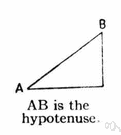# hypotenuse

Also found in: Thesaurus, Medical, Acronyms, Encyclopedia, Wikipedia.

## hy·pot·e·nuse

(hī-pŏt′n-o͞os′, -yo͞os′) also hy·poth·e·nuse (-pŏth′ə-no͞os′, -nyo͞os′)
n. Abbr. hyp.
The side of a right triangle opposite the right angle.

[Latin hypotēnūsa, from Greek hupoteinousa, from feminine present participle of hupoteinein, to stretch or extend under : hupo-, hypo- + teinein, to stretch; see ten- in Indo-European roots.]

## hypotenuse

(haɪˈpɒtɪˌnjuːz)
n
(Mathematics) the side in a right-angled triangle that is opposite the right angle. Abbreviation: hyp
[C16: from Latin hypotēnūsa, from Greek hupoteinousa grammē subtending line, from hupoteinein to subtend, from hypo- + teinein to stretch]

## hy•pot•e•nuse

(haɪˈpɒt nˌus, -ˌyus)

n.
the side of a right triangle opposite the right angle.
[1565–75; < Latin hypotēnūsa < Greek hypoteínousa (grámmē) subtending (line), feminine present participle of hypoteínein to subtend =hypo- hypo- + teínein to stretch (see thin)]

## hy·pot·e·nuse

(hī-pŏt′n-o͞os′)
The side of a right triangle opposite the right angle. It is the longest side, and the square of its length is equal to the sum of the squares of the lengths of the other two sides.
ThesaurusAntonymsRelated WordsSynonymsLegend:
 Noun 1hypotenuse - the side of a right triangle opposite the right angleright triangle, right-angled triangle - a triangle with one right angleflank - a subfigure consisting of a side of something
Translations
hipotenusa
přepona
hypotenuusa
hipotenuza
langhlið

hypotenusa

## hypotenuse

[haɪˈpɒtɪnjʊːz] N (Math) →

## hypotenuse

[haɪˈpɒtənjuːz] n

## hypotenuse

nHypotenuse f; the square on the hypotenusedas Quadrat über der Hypotenuse

## hypotenuse

[haɪˈpɒtɪnjuːz] n (Geom) → ipotenusa
References in periodicals archive ?
Caption: FIG 3: Schematic Illustration of Viscoelastic Properties: The complex modulus (or Young's modulus) can be thought of as the hypotenuse of a right triangle, while the elastic and viscous modulus represent the other two sides.
a Right Angled###* when hypotenuse and the vertical height from its vertex
Several isolated spread concrete foundation slabs with different width-to-height ratios and hypotenuse slopes were tested under the uplift load firstly.
Caption: Imagine a right triangle that uses the line that runs between Mizar and Alioth in Ursa Major as the hypotenuse. The Eiffel Tower asterism occupies the 90[degrees] corner of the triangle.
For example, in finding the hypotenuse of a right angle triangle using c = [square root of (([a.sup.2] + [b.sup.2]))], we can discuss how a, b and c are variables, what they mean and which are the independent variables (in this case two of them) and which is the dependent variable.
Any point on the hypotenuse UV is associated with x(t, [gamma]), x(t, [gamma]), where [[gamma].sub.*] < [gamma] [less than or equal to] [[gamma].sup.*].
Observations such as "this game of darts is twisting like a rattlesnake with a hernia" were supplemented with well-planned lines like "there's no one quicker than these two tungsten tossers" and, a personal favourite, "even Hypotenuse would have trouble working out these angles."
Observations such as, "This game of darts is twisting like a rattlesnake with a hernia," were supplemented with well-planned lines like, "There's no one quicker than these two tungsten tossers," and, a personal favourite, "Even Hypotenuse would have trouble working out these angles."
Oh, and when I get to the Pearly Gates, Mr Pythagoras better do a runner before he gets a boot in the hypotenuse.
Like other languages, it has its own vocabulary that includes words found only in mathematics, such as "hypotenuse" and "trapezoid," as well as common words that have distinct meanings when used in a math-specific context, such as "table" and "median."
The fixed shape of the Hayekian triangle means that the slope of the Hayekian triangle's hypotenuse is fixed.
From memory, I sat at the back, looking out of the window coming up with various career choices in which I would never need to say the word, or even understand the meaning of hypotenuse.

Site: Follow: Share:
Open / Close